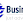## Count left right node of a MLM(Multi Lavel marketing) binary tree with database in ASP.NET C# - double recursion

Here I will show how to count the number of left right node of a parent node or some node.

Here I am sueing MS. SQL Database and structure is some thing like this.

-------------------------------------------------------
Id(int) | Parent_Id (int) | Placement (NVarChar(10))
-------------------------------------------------------
1          0                        -
2          1                        Left
3          1                        Right
4          2                        Left
5          2                        Right
-------------------------------------------------------

Here I am using double recursion to find the count of left & right node.

Code:
`/* Call these from any method */`
```Data obj = new Data();
pNodeL(LeftNode, "");
pNodeR(RightNode, "");

/// <summary>
/// To Count right node
/// </summary>
/// <param name="node"></param>
/// <param name="place"></param>
private void pNodeR(string node, string place)
{
string sql = "select * from biodata_master where parent_no='" + node + "'";

DataTable dt = new DataTable();
dt = obj.SelectData(sql);

if (dt.Rows.Count == 1)
{
Cr = Cr + 1;
}
else if (dt.Rows.Count > 0)
{
Cr = Cr + 2;
pNodeR(dt.Select("PLACEMENT='Left'").ItemArray.ToString(), "LEFT");
pNodeR(dt.Select("PLACEMENT='Right'").ItemArray.ToString(), "RIGHT");
}
}

/// <summary>
/// To Count left node
/// </summary>
/// <param name="node"></param>
/// <param name="place"></param>
private void pNodeL(string node,string place)
{
string sql = "select * from biodata_master where parent_no='"+node+"'";

DataTable dt = new DataTable();
dt = obj.SelectData(sql);

if (dt.Rows.Count == 1)
{
Cl = Cl + 1;
}
else if (dt.Rows.Count > 1)
{
Cl = Cl + 2;
pNodeL(dt.Select("PLACEMENT='Left'").ItemArray.ToString(), "LEFT");
pNodeL(dt.Select("PLACEMENT='Right'").ItemArray.ToString(), "RIGHT");
}
}

/* SelectData Method & Connection string */

SqlConnection con = new SqlConnection(WebConfigurationManager.ConnectionStrings["ConnectionString"].ToString());

public System.Data.DataTable SelectData(string sql)
{
try
{
DataTable dt = new DataTable();
da.Fill(dt);
return dt;
}
catch
{
throw;
}
}```

It will return your left & right count in the variable Cl and Cr.
Try yours.

1.The name 'obj' does not exist in the current context
The name 'Cr' does not exist in the current context

Generating flowing errors

2.Unable to find DATA class..

3.Please, can these code be translated in PHP MYSQL.

4.Sir jee Wll you give me source code of above code

5.